## Part of Speech Tagging

• What is part of speech tagging
• Markov chains
• Hidden Markov models
• Viterbi models

Part-of-speech refers to the category of words or the lexical terms in the language. Examples of these lexical terms in the English language would be noun, verb, adjective, adverb, pronoun, preposition, although there are many others.

## Markov Chains

Markov property: the probability of the next event only depends on the current events.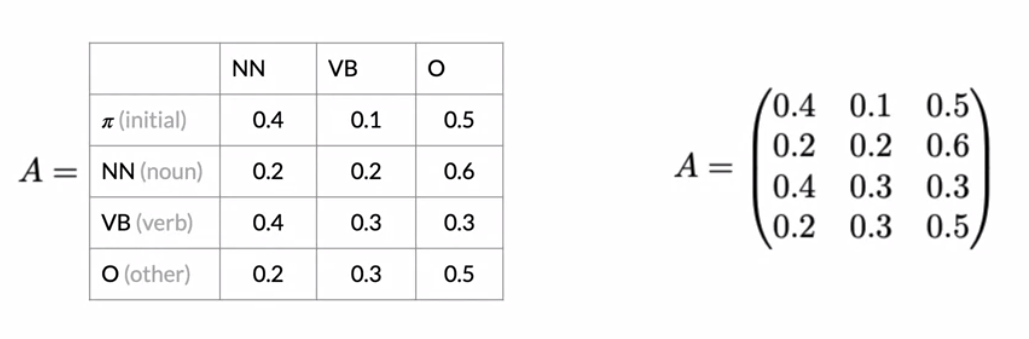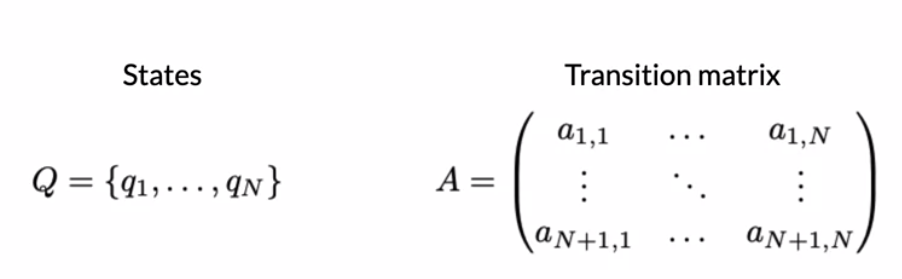Emission probabilities are the probabilities to the observables, the words in the input.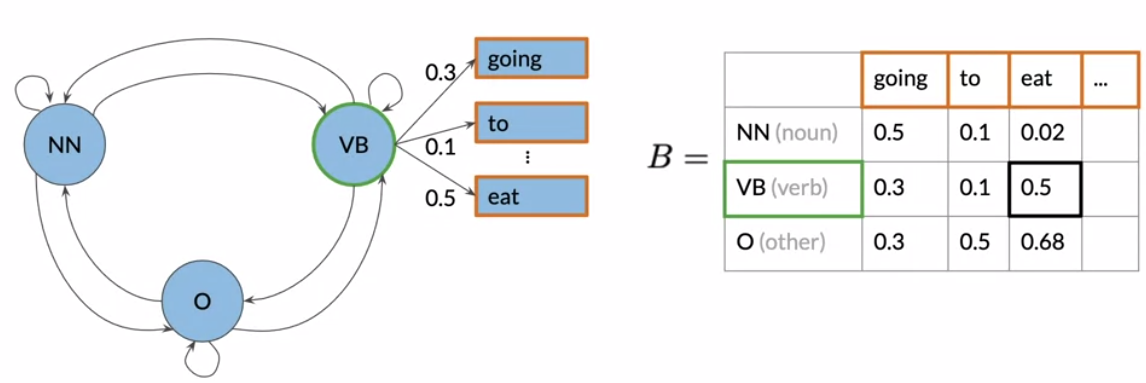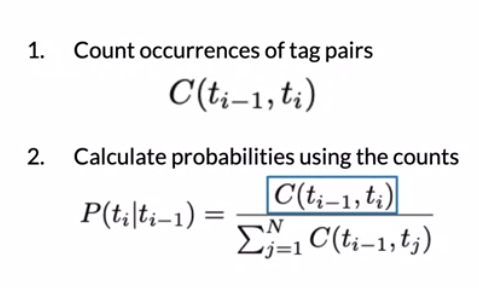### Populating the Transition Matrix

Smoothing can be added to avoid zero division and help generalization. Smoothing can’t be done with the first row $\pi$, tough, as it could allow the sentence to start with punctiation, for example.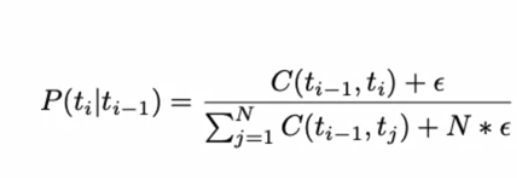### Populating the Emission Matrix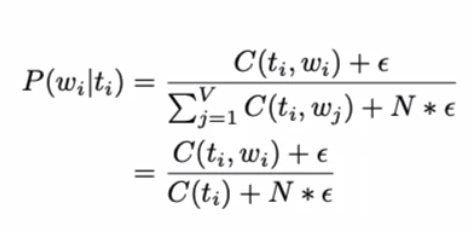## The Viterbi Algorithm

It’s a graph algorithm.

### Initialization step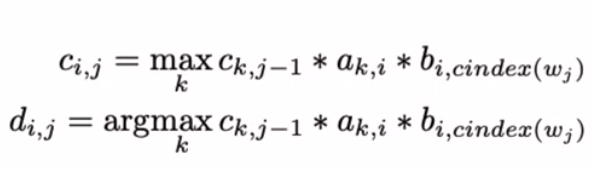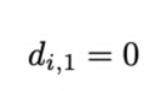### Forward pass### Backward pass

Calculate the index of the biggest probability in the last step and backward get the steps accordingly in the D matrix.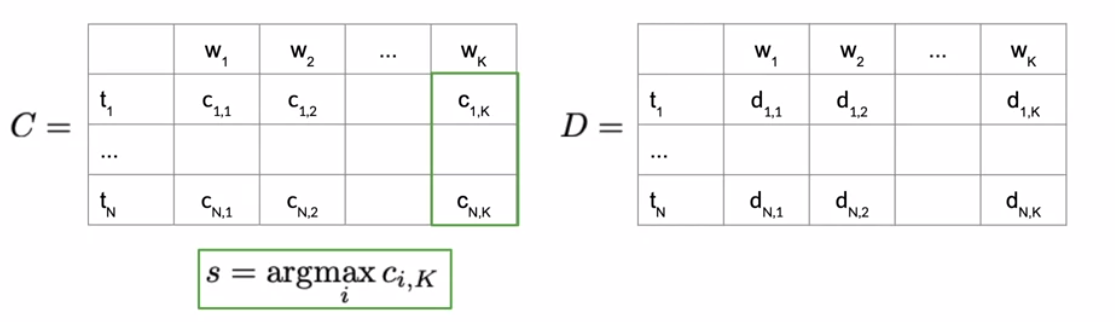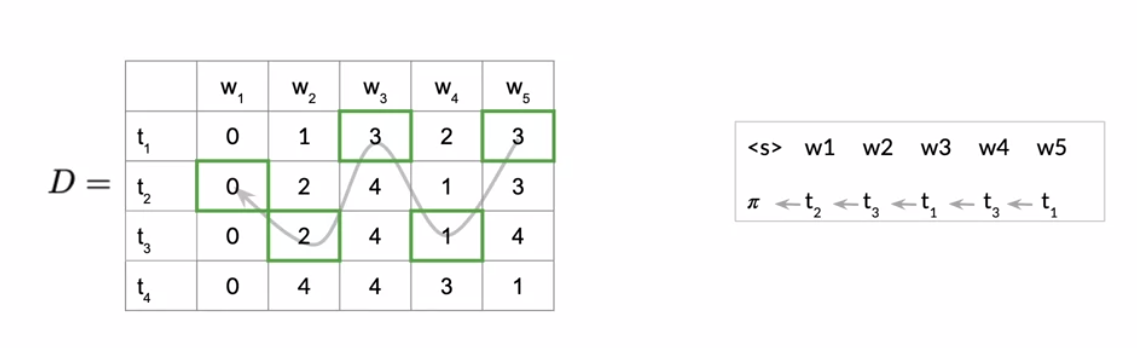Log probabilities help the problem of consecutive multiplication of small numbers.